# Absorption of quantum wells¶

For modelling the optical properties of QWs we use the method described by S. Chuang (). The absorption coefficient at thermal equilibrium in a QW is given by: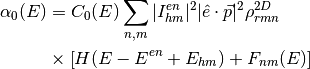where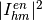is the overlap integral between the holes in level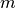and the electrons in level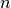;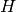is a step function,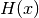= 1 for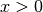, 0 and 0 for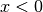,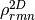is the 2D joint density of states,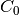a proportionality constant dependent on the energy, and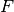the excitonic contribution, which will be discussed later.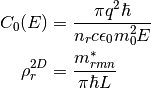Here,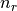is the refractive index of the material,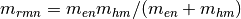the reduced, in-plane, effective mass and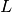an effective period of the quantum wells. The in-plane effective mass of each type of carriers is calculated for each level, accounting for the spread of the wavefunction into the barriers as ():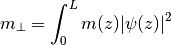This in-plane effective mass is also used to calculate the local density of states shown in Figure [fig:qw]b. In Eq. [eq:QW_abs2],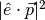is the momentum matrix element, which depends on the polarization of the light and on the Kane’s energy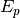, specific to each material and determined experimentally. For band edge absorption, where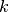= 0, the matrix elements for the absorption of TE and TM polarized light for the transitions involving the conduction band and the heavy and light holes bands are given in Table [tab:matrix_elements]. As can be deduced from this table, transitions involving heavy holes cannot absorb TM polarised light.

TE TM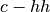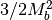0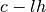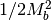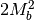Table: Momentum matrix elements for transitions in QWs.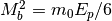is the bulk matrix element.

In addition to the band-to-band transitions, QWs usually have strong excitonic absorption, included in Eq. [eq:qw_abs] in the term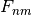. This term is a Lorenzian (or Gaussian) defined by an energy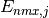and oscillator strength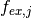. It is zero except for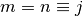where it is given by Klipstein et al. ():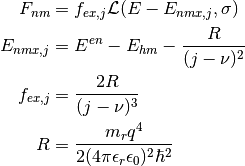Here,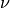is a constant with a value between 0 and 0.5 and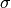is the width of the Lorentzian, both often adjusted to fit some experimental data. In Solcore, they have default values of= 0.15 and= 6 meV.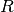is the exciton Rydberg energy ().

Fig. [fig:QW_absorption] shows the absorption coefficient of a range of InGaAs/GaAsP QWs with a GaAs interlayer and different In content. Higher indium content increases the depth of the well, allowing the absorption of less energetic light and more transitions.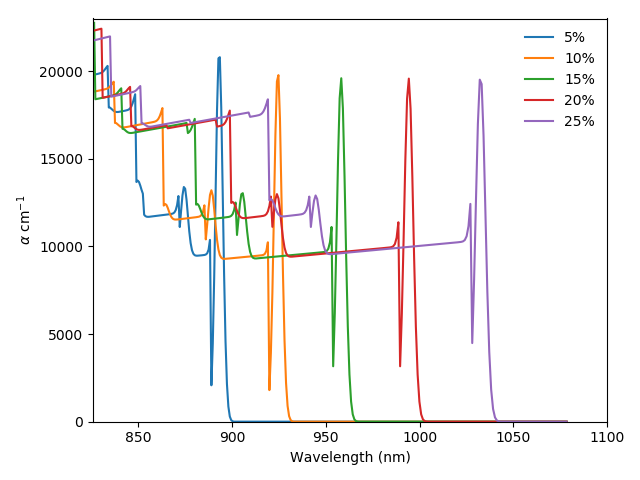solcore.absorption_calculator.absorption_QW.exciton_rydberg_energy_2d(me, mh, eps_r)[source]
Parameters: me – electron effective mass (units: kg) mh – hole effective mass (units: kg) eps_r – dielectic constant (units: SI) The exciton Rydberg energy
solcore.absorption_calculator.absorption_QW.exciton_bohr_radius(me, mh, eps)[source]
Parameters: me – electron effective mass (units: kg) mh – hole effective mass (units: kg) eps – dielectic constant (units: SI) Exciton Borh radius
solcore.absorption_calculator.absorption_QW.alpha_c_hh_TE(E, z, E_e, E_hh, psi_e, psi_hh, well_width, me, mhh, Ep, nr)[source]

Absortion coefficient for incident light forming a transision between hh and c band of a quantum well

NB. Assumes that valence band is a zero energy, might need to manually apply an offset.

Parameters: E – photon energy (units: J) z – mesh points along growth direction (units: m) E_e – Electron state energy (units: J) E_hh – Heavy hole state energy (units: J) psi_e – Electron envelope function (psi_e^2 must be normalised) psi_hh – Heavy hole envelope function (psi_hh^2 must be normalised) well_width – (units: m) me – electron effective mass (units: kg) mhh – heavy hole effective mass (units: kg) Ep – the Kane parameter “Optical dipole matrix elemet”, sometimes “Momentum matrix element”, e.g. Ep for GaAs ~ 28eV nr – refractive index
solcore.absorption_calculator.absorption_QW.alpha_c_lh_TE(E, z, E_e, E_lh, psi_e, psi_lh, well_width, me, mlh, Ep, nr)[source]

Absortion coefficient for incident light forming a transision between hh and c band of a quantum well

NB. Assumes that valence band is a zero energy, might need to manually apply an offset.

Parameters: E – photon energy (units: J) z – mesh points along growth direction (units: m) E_e – Electron state energy (units: J) E_hh – Heavy hole state energy (units: J) psi_e – Electron envelope function (psi_e^2 must be normalised) psi_hh – Heavy hole envelope function (psi_hh^2 must be normalised) well_width – (units: m) me – electron effective mass (units: kg) mhh – heavy hole effective mass (units: kg) Ep – the Kane parameter “Optical dipole matrix elemet”, sometimes “Momentum matrix element”, e.g. Ep for GaAs ~ 28eV nr – refractive index
solcore.absorption_calculator.absorption_QW.alpha_exciton_ehh_TE(exciton_index, E, z, E_e, E_hh, psi_e, psi_hh, well_width, me, mhh, Ep, nr, eps, hwhm=9.6e-22, dimensionality=0.15, line_shape='Lorenzian')[source]
Parameters: exciton_index – E – z – E_e – E_hh – psi_e – psi_hh – well_width – me – mhh – Ep – nr – eps – hwhm – dimensionality – line_shape –
solcore.absorption_calculator.absorption_QW.alpha_exciton_elh_TE(exciton_index, E, z, E_e, E_lh, psi_e, psi_lh, well_width, me, mlh, Ep, nr, eps, hwhm=9.6e-22, dimensionality=0.15, line_shape='Lorenzian')[source]
Parameters: exciton_index – E – z – E_e – E_lh – psi_e – psi_lh – well_width – me – mlh – Ep – nr – eps – hwhm – dimensionality – line_shape –
solcore.absorption_calculator.absorption_QW.sum_alpha_c_hh_TE(E, z, E_e, E_hh, psi_e, psi_hh, well_width, me, mh, Ep, nr)[source]
Parameters: E – z – E_e – E_hh – psi_e – psi_hh – well_width – me – mh – Ep – nr –
solcore.absorption_calculator.absorption_QW.sum_alpha_c_lh_TE(E, z, E_e, E_lh, psi_e, psi_lh, well_width, me, mh, Ep, nr)[source]
Parameters: E – z – E_e – E_lh – psi_e – psi_lh – well_width – me – mh – Ep – nr –
solcore.absorption_calculator.absorption_QW.sum_alpha_exciton_c_hh_TE(E, z, E_e, E_hh, psi_e, psi_hh, well_width, me, mh, Ep, nr, eps, hwhm=9.6e-22, dimensionality=0.5, line_shape='Lorenzian')[source]
Parameters: E – z – E_e – E_hh – psi_e – psi_hh – well_width – me – mh – Ep – nr – eps – hwhm – dimensionality – line_shape –
solcore.absorption_calculator.absorption_QW.sum_alpha_exciton_c_lh_TE(E, z, E_e, E_lh, psi_e, psi_lh, well_width, me, mlh, Ep, nr, eps, hwhm=9.6e-22, dimensionality=0.5, line_shape='Lorenzian')[source]
Parameters: E – z – E_e – E_lh – psi_e – psi_lh – well_width – me – mlh – Ep – nr – eps – hwhm – dimensionality – line_shape –
solcore.absorption_calculator.absorption_QW.calc_alpha(QM_result, well_width, kane_parameter=4.48e-18, refractive_index=3.5, hwhm=9.6e-22, dimensionality=0.5, theta=0, eps=1.1421902283930001e-10, espace=None, line_shape='Lorenzian')[source]

Calculates the absorption coeficient of a quantum well structure assuming the parabolic approximation for the effective masses.

Parameters: QM_result – The output of the Schrodinger solver, incldued in the ‘quantum_mechanics’ package well_width – The well width kane_parameter – The Kane parameter refractive_index – Refractive (effective) index of the QW hwhm – Full width at half maximum of the excitonic lineshape dimensionality – theta – eps – espace – line_shape –
solcore.absorption_calculator.absorption_QW.NonBlackBodyEmission(E, voltage=0, nr=3.5, T=300)[source]
Parameters: E – voltage – nr – T –
solcore.absorption_calculator.absorption_QW.calc_emission(QM_result, well_width, voltage=0, theta=0)[source]
Parameters: QM_result – well_width – voltage – theta –